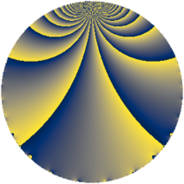# Properties

 Label 432.1.eLevel $432$ Weight $1$ Character orbit 432.e Rep. character $\chi_{432}(161,\cdot)$ Character field $\Q$ Dimension $1$ Newform subspaces $1$ Sturm bound $72$ Trace bound $0$

# Related objects

## Defining parameters

 Level: $$N$$ $$=$$ $$432 = 2^{4} \cdot 3^{3}$$ Weight: $$k$$ $$=$$ $$1$$ Character orbit: $$[\chi]$$ $$=$$ 432.e (of order $$2$$ and degree $$1$$) Character conductor: $$\operatorname{cond}(\chi)$$ $$=$$ $$3$$ Character field: $$\Q$$ Newform subspaces: $$1$$ Sturm bound: $$72$$ Trace bound: $$0$$

## Dimensions

The following table gives the dimensions of various subspaces of $$M_{1}(432, [\chi])$$.

Total New Old
Modular forms 22 1 21
Cusp forms 4 1 3
Eisenstein series 18 0 18

The following table gives the dimensions of subspaces with specified projective image type.

$$D_n$$ $$A_4$$ $$S_4$$ $$A_5$$
Dimension 1 0 0 0

## Trace form

 $$q + q^{7} + O(q^{10})$$ $$q + q^{7} - q^{13} + q^{19} + q^{25} - 2 q^{31} - q^{37} - 2 q^{43} - q^{61} + q^{67} - q^{73} + q^{79} - q^{91} - q^{97} + O(q^{100})$$

## Decomposition of $$S_{1}^{\mathrm{new}}(432, [\chi])$$ into newform subspaces

Label Dim. $$A$$ Field Image CM RM Traces $q$-expansion
$$a_2$$ $$a_3$$ $$a_5$$ $$a_7$$
432.1.e.a $$1$$ $$0.216$$ $$\Q$$ $$D_{3}$$ $$\Q(\sqrt{-3})$$ None $$0$$ $$0$$ $$0$$ $$1$$ $$q+q^{7}-q^{13}+q^{19}+q^{25}-2q^{31}+\cdots$$

## Decomposition of $$S_{1}^{\mathrm{old}}(432, [\chi])$$ into lower level spaces

$$S_{1}^{\mathrm{old}}(432, [\chi]) \cong$$ $$S_{1}^{\mathrm{new}}(108, [\chi])$$$$^{\oplus 3}$$